In this video, we are going to look at area sector and arc length.

Here we have two radii drawn from circle O that are perpendicular to each other, so that the angle formed is a right angle. That is one-fourth of the whole circle. If we wanted to find the shaded area, we would expect it to be one-fourth of the entire area of the whole circle. If we had a diameter drawn instead, then the new shaded area would represent half of the area of the circle. There is a direct relationship between the angle and the area of the circle. This relationship is a proportion that exists between the angle that we have,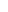$\theta$, and the area of the shaded region,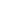$S$. The proportion is: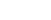$\frac{\theta}{360}=\frac{S}{A}$

For example:$\frac{90}{360}=\frac{S}{A}$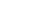$\frac{1}{4}=\frac{S}{A}$
This means that this shaded area,$S$, is one-fourth of the area of the entire circle.

For example:
If we had an angle that was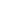$40\textdegree$ and the radius is$6$ inches, then how would we solve this? Well, if we plug in our angles measure into our proportion, we have:$\frac{40}{360}=\frac{S}{A}$

In order to calculate S, we first need to know the total area. We need to use our area of a circle formula: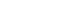$A=\pi{r^2}$$A=\pi{6^2}$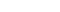$A=36\pi$

So: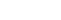$\frac{40}{360}=\frac{S}{36\pi}$

We can first reduce this to: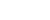$\frac{1}{9}=\frac{S}{36\pi}$

Now we can cross multiply: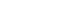$36\pi=9S$
Divide both sides by 9: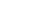$4\pi=S$

We can also use the angle measure to help us find the arc length. Just like how the shaded area was a fraction of the total area, the arc is a fraction of the total circumference. This formula is: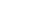$\frac{\theta}{360}=\frac{L}{C}$

For example:
If we had an angle that was$40\textdegree$ and the radius is$6$ inches, then how would we solve this? Well, if we plug in our angles measure into our proportion, we have:$\frac{40}{360}=\frac{L}{C}$

In order to calculate L, we first need to know the circumference. We need to use our circumference formula: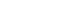$C=2\pi{r}$$C=2\pi{6}$$C=12\pi$

So: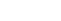$\frac{40}{360}=\frac{L}{12\pi}$

We can first reduce this to: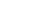$\frac{1}{9}=\frac{L}{12\pi}$

Now we can cross multiply: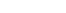$12\pi=9L$

Divide both sides by 9: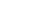$\frac{4\pi}{3}=L$